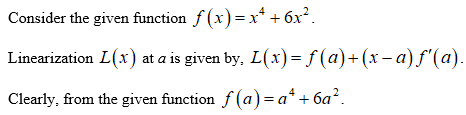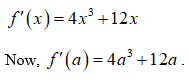# Find the linearization L(x) of the function at a.f(x) = x4 + 6x2, a = −1

Question
15 views

Find the linearization L(x) of the function at a.

f(x) = x4 + 6x2, a = −1
check_circle

Step 1Step 2

Differentiate the given function with respect to x.Step 3

The linearization at a = ­–1...

### Want to see the full answer?

See Solution

#### Want to see this answer and more?

Solutions are written by subject experts who are available 24/7. Questions are typically answered within 1 hour.*

See Solution
*Response times may vary by subject and question.
Tagged in

### Derivative